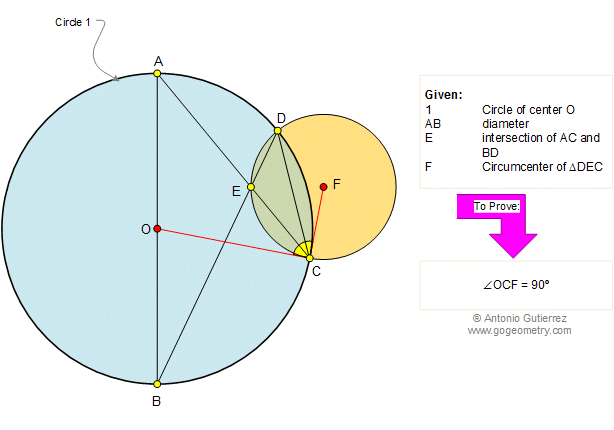# Defining Geometry, Branches, and Formula of the ConceptDo you know what the term ‘Geometry’ means? It actually means ‘measurement of the earth’ (‘Geo’ means ‘earth’ while ‘metry’ is ‘measurement’ in Greek. In the study of Geometry, we measure different things and objects which are of varied shapes and structures. Some earthly structures are circles like the wall clock, cylindrical like the gas cylinder, or rectangles like the shape of your cell phone. So, measuring these earthly structures while estimating their length, breadth, area, or perimeter we need the application of Geometry. Thus, we now see how it got its name.

Before studying Geometry, there are various forms of Geometry. In plane geometry, there are 2-dimensional structures like the triangles or the squares; this measuring of the 2d structures is called the plane geometry. There is another variety of Geometry, which is known as the ‘Solid geometry’ which measures three-dimensional structures like the cube, cuboid, or cone-like shapes. The last geometry types are the ‘basic geometry’ which is focused on the planes or line shapes.

These various types of Geometry actually allow us to have measured a wide range of shapes in our earthly life.

## How Would You Define Geometry?

In the purview of Geometry, we learn about different shapes and structures. With the help of this subject, first, as a child, we identify what is a line, or a circle, or a square. Then as we proceed to grow, we learn how to estimate the measurements of these structures. We learn about the formulae and the measurements which help us in estimating the volume, perimeter, and other aspects of the measurement. Next, as we start our career in the field of engineering or other related work in geometry, we apply the study in our work. So, we see not only as a subject but in life as a totality, geometry teaches us about different forms and measurements.

## Branches of Geometry

The branches of the study of geometry can be categorized as under:

• Algebraic geometry
• Discrete geometry
• Differential geometry
• Euclidean geometry
• Convex geometry
• Topology

### What are Geometry Formulas?

In the study of Geometry, in order to calculate different measurements of the related shapes, there is the use of length, breadth, and height of the different geometrical figures. These all together form the geometrical formulae. Thus, there are abundant formulae to learn in geometry that will facilitate each and every aspect of measuring.

## Horizontal Line Definition

The horizontal line is nothing but simply a line drawn from left to right or right to left. This line goes from right to left. In the coordinate type geometry, this line is said to lie on the horizon. Suppose there are two points placed side by side, then the line which is joining this point is called the horizontal line. If drawn in a graph, then a horizontal line is always parallel to the x-axis. While a vertical line will always be parallel to the Y-axis. The Horizontal line will be touching the Y-axis and forming a parallel line to the X-axis.

### What is the Slope of the Horizontal line?

y = MX + c and y = km = 0

After comparing the slope-intercept form of the equation, we get the slope of the horizontal line which is always equal to the number zero.

## Steps of Drawing a Horizontal Line

The steps of drawing a horizontal line are as follows:

• First, know the two pints, suppose a and b
• Now place the points a and b after each other on a PQ plane.
• Now in the graph paper draw a line parallel to X-axis touching a and b
• You get your horizontal line here.

Thus, it is so easy to draw the horizontal line. As we come to the end of the content, the students are now having a good understanding of Geometry and Horizontal line. Visit Cuemath for more interesting and important concepts.